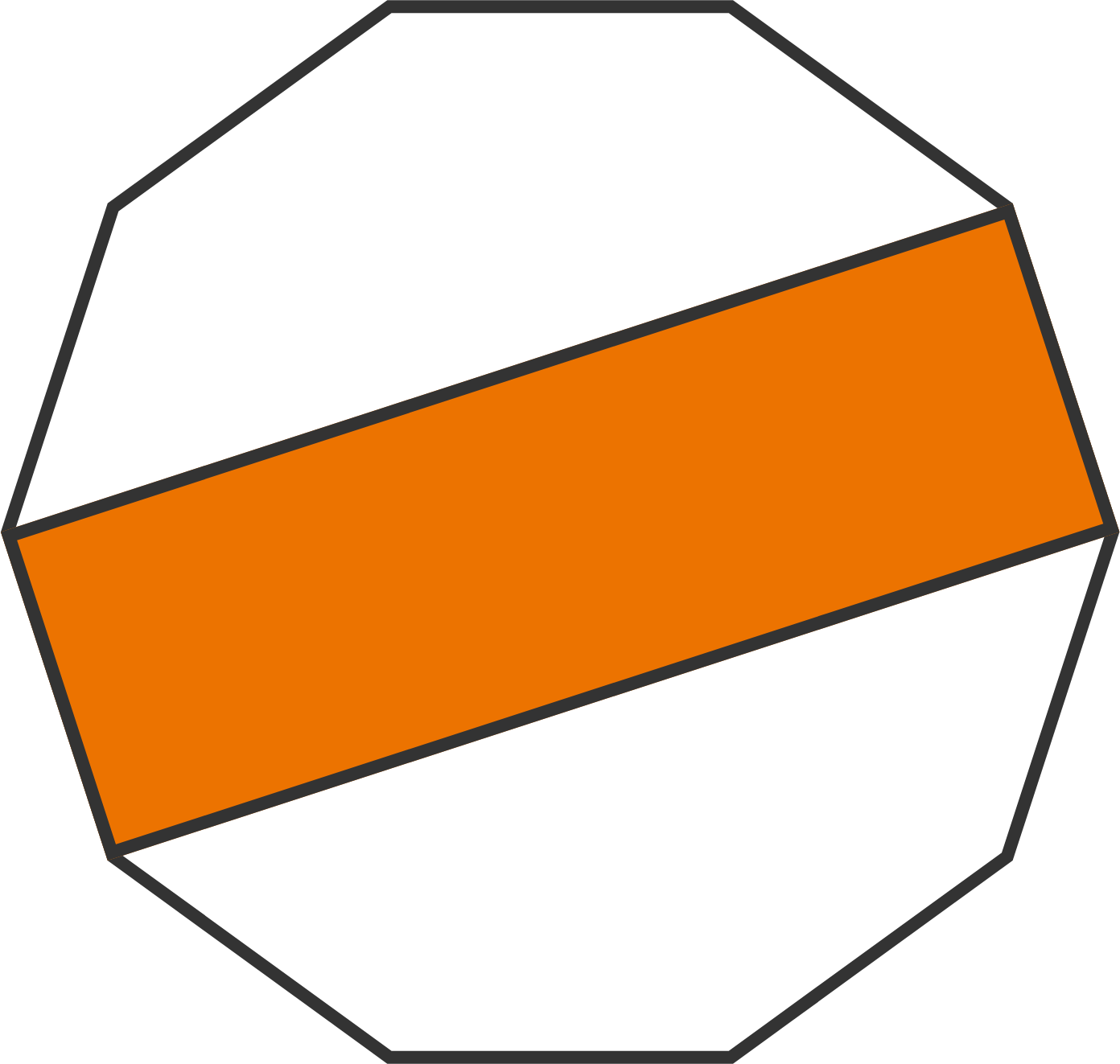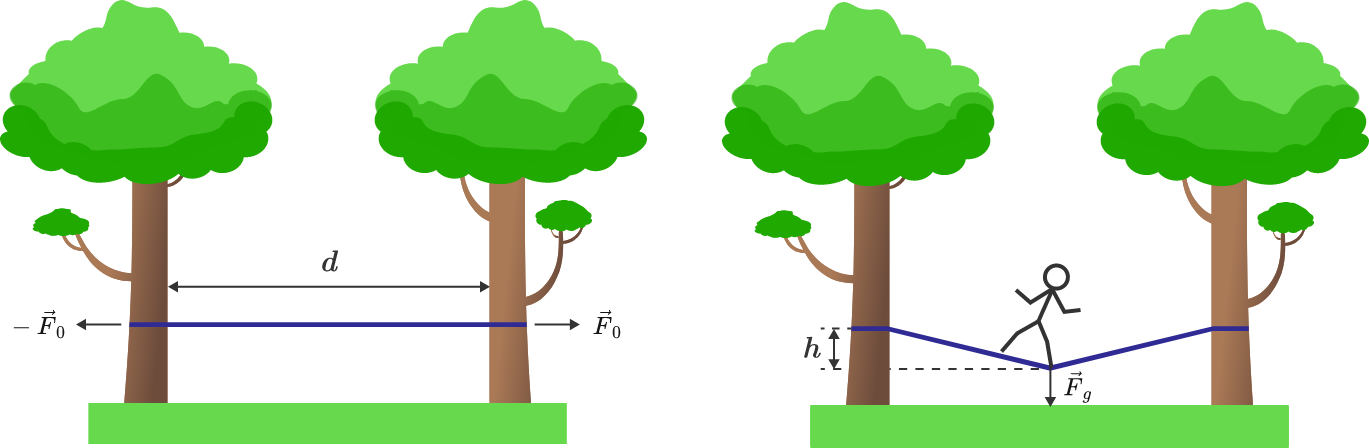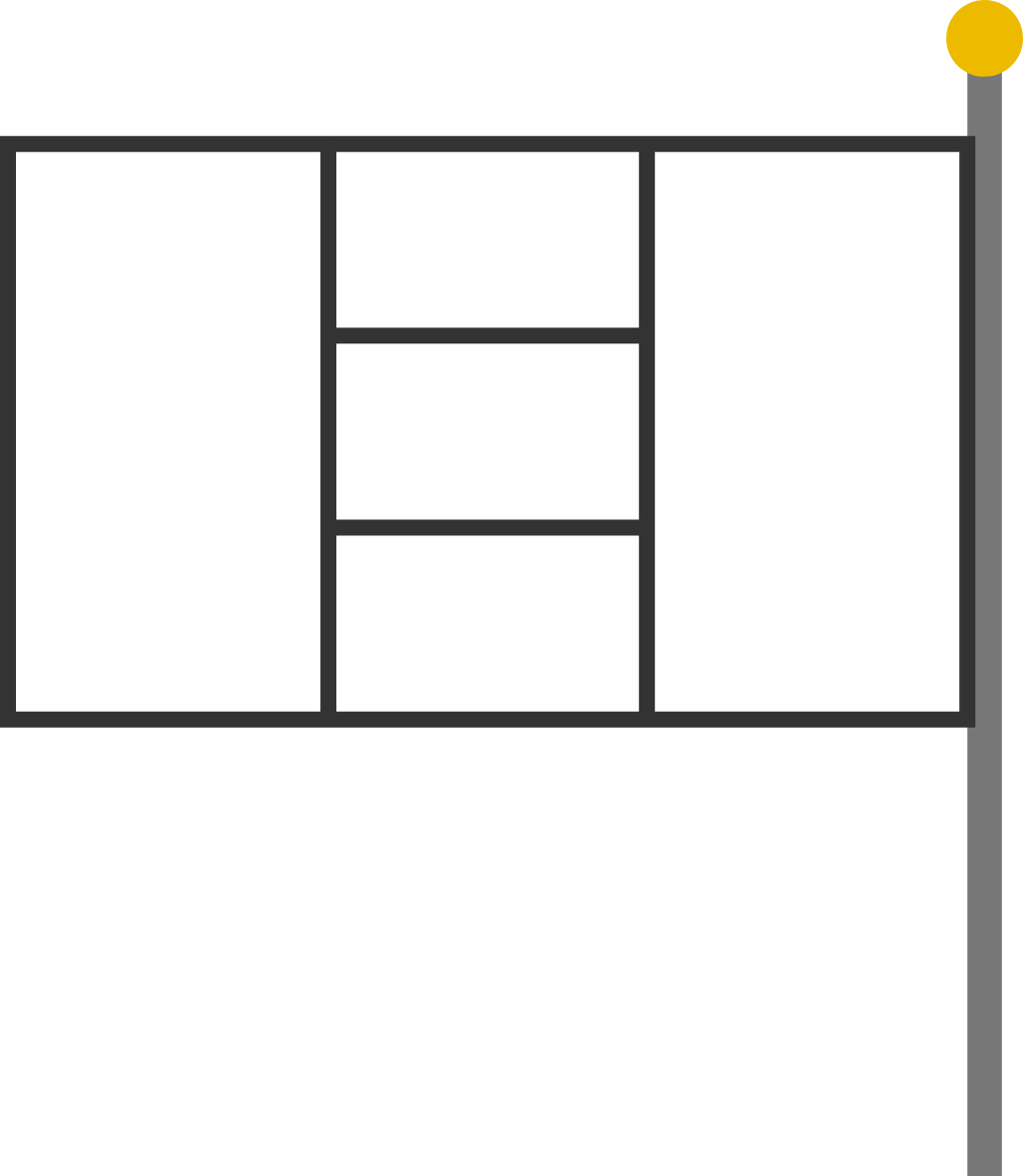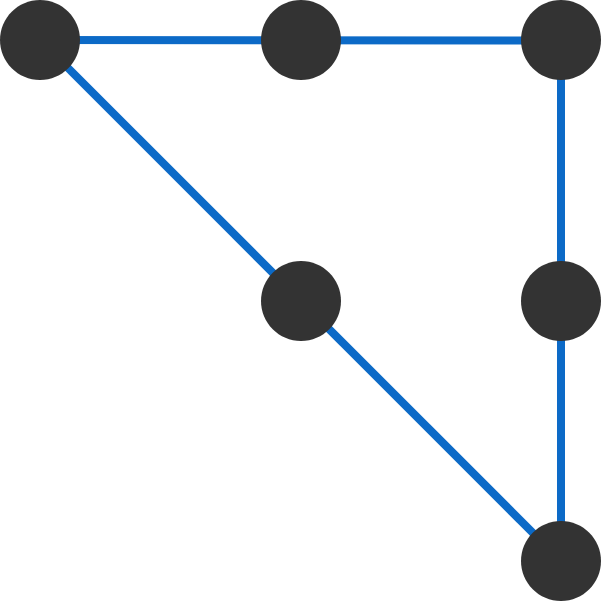# Problems of the Week

Contribute a problem

# 2017-12-11 Intermediate

In the regular decagon below, find the percentage of area that is shaded orange.$ab = a^2+b^2$ It is obvious that $a=b=0$ satisfies the equation above. But is there another pair of real numbers $(a,b)$ satisfying this equation?

A slackline is stretched between two trees at a distance, $d = 10\,\text{m}$, so that a tensioning force, $F_0 = 5000 \,\text{N}$, is applied on the empty rope. A man then balances in the middle of the rope and exerts a weight force, $F_g = 750 \,\text{N}$, onto the slackline. What is the vertical deflection, $h$, of the rope?Details and Assumptions: The rope is made of an elastic material and obeys Hooke's law $F = K \frac{l - l_0}{l_0},$ where $F$ is the force along the rope, $l_0$ is the initial length, $l$ is the stretched length of the rope, and $K = 150\text{ kN}$ is the force constant.You have a set of 10 paints of distinct colors and a flag with 5 regions, shown to the right.

In how many ways can you color all 5 of the regions such that regions that share an edge are different colors?


Note: The flag is attached to a flagpole, which prevents the flag from being rotated or flipped.

What is the minimum number of soldiers needed to form $12$ lines of at least $3$ soldiers each?6 soldiers can form 3 lines of 3 soldiers each


Note: The lines must be distinct. For example, if you have 5 soldiers in a line, this only counts as 1 line.

×